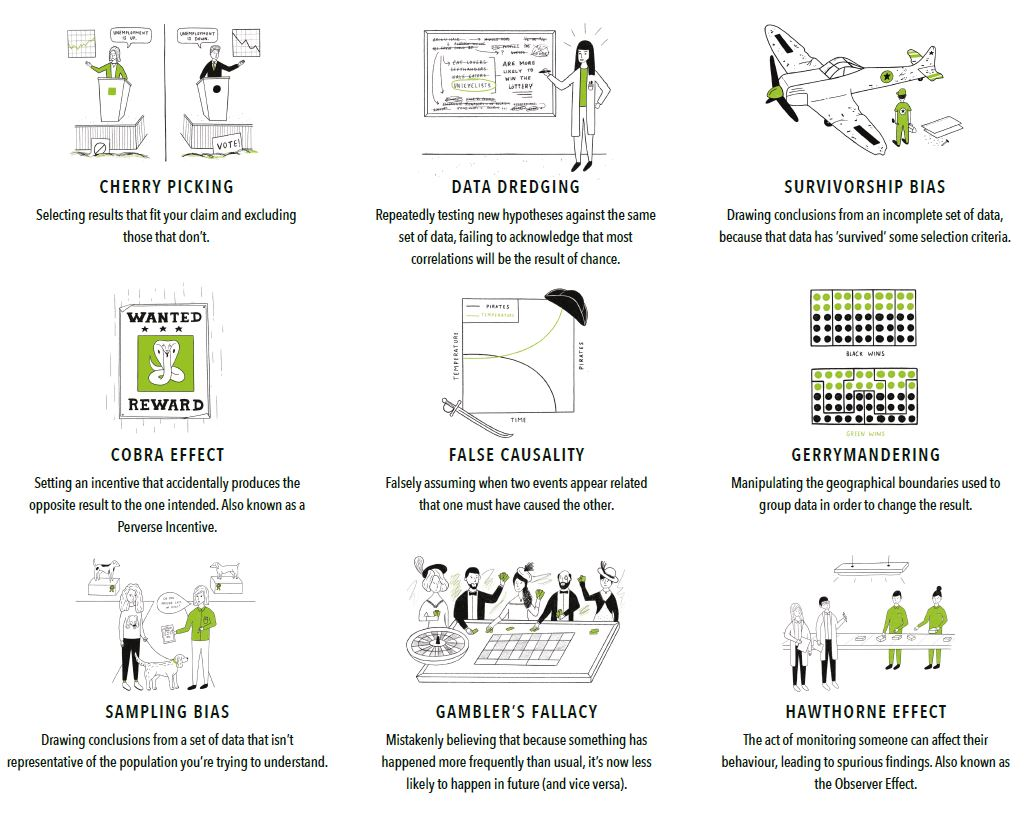# 5.2.5. Statistical significance¶

Previously, we estimated

$\log(\text{price})= 8.2+ 1.53 *\log(\text{carat}) + 0.33* \text{Ideal} + 0.18* \log(\text{carat})\cdot \text{Ideal}$

Those coefficients are estimates, not gospel. There is some uncertainty about what the “true” value should be.# load some data to practice regressions
import seaborn as sns
import numpy as np
from statsmodels.formula.api import ols as sm_ols # need this

# this alteration is not strictly necessary to practice a regression
# but we use this in livecoding
diamonds2 = (diamonds.query('carat < 2.5')               # censor/remove outliers
.assign(lprice = np.log(diamonds['price']))  # log transform price
.assign(lcarat = np.log(diamonds['carat']))  # log transform carats
.assign(ideal = diamonds['cut'] == 'Ideal')

# some regression packages want you to explicitly provide
# a variable for the constant
.assign(const = 1)
)

(
sm_ols('lprice ~ lcarat + ideal + lcarat*ideal',
data=diamonds2.query('cut in ["Fair","Ideal"]'))
).fit().summary().tables # the summary is multipe tables stitched together. let's only print the params

coef std err t P>|t| [0.025 0.975] 8.1954 0.007 1232.871 0.000 8.182 8.208 0.3302 0.007 46.677 0.000 0.316 0.344 1.5282 0.015 103.832 0.000 1.499 1.557 0.1822 0.015 12.101 0.000 0.153 0.212

Thus, the table that regression produces (which you can see above by clicking the plus button) also displays information next to each coefficient about the level of precision associated with the estimated coefficients.

Column

Meaning

“std err”

The standard error of the coefficient estimate

“t”

The “t-stat” = $$\beta$$ divided by its standard error

“P>t”

The “p-value” is the probability that the coefficient is different than zero by random chance.

“[0.025 0.975]”

The 95% confidence interval for the coefficient.

Note

We use these columns, particularly the “t-stat” and “p-value”, to assess the probability that the coefficient is different than zero by random chance.

• A t-stat of 1.645 corresponds to a p-value of 0.10; meaning only 10% of the time would you get that coefficient randomly

• A t-stat of 1.96 corresponds to a p-value of 0.05; this is a common “threshold” to say a “relationship is statistically significant” and that “the relationship between X and y is not zero”

• A t-stat of 2.58 corresponds to a p-value of 0.01

The “classical” approach to assess whether X is related to y is to see if the t-stat on X is above 1.96. These thresholds are important and part of a sensible approach to learning from data, but…

When people say a “relationship is statistically significant”, what they often mean is

"LOOK YONDER, I HAVE FOUND A NEW, MEANINGFUL
CORRELATION THAT IS TRUE
(TRULY!) (VERILY!)
AND KNOWING IT ...
WILL CHANGE THE WORLD!"

Which leads me to the next section.

## 5.2.5.1. Significant warnings about “statistical significance”¶

The focus on t-stats p-values can be dangerous. As it turns out, “p-hacking” (finding a significant relationship) is easy for a number of reasons:

• If you look at enough Xs and enough ys, you, by chance alone, can find “significant” relationships where none exist

• Your data can be biased. Dewey Defeats Truman may be the most famous example• Your sample restrictions can generate bias: If you evaluate the trading strategy “buy and hold current S&P companies” for the last 50 years, you’ll discover that this trading strategy was unbelievable!

• Reusing the data: If you torture the data, it will confess!

There is a fun game in here.

The point:

1. The choices you make about what variables to include or focus on can change the statistical results.

2. **And if you play with a dataset long enough, you’ll find “results”… ***

### 5.2.5.1.1. The focus on p-values can be dangerous because it distorts the incentives of analysts¶

• Again: If you torture the data, it will confess (regardless of whether you or it wanted to)

• Motivated reasoning/cognitive dissonance/confirmation bias: Analysis like the game above is fraught with temptations for humans. That 538 article mentions that about 2/3 of retractions are due to misconduct.

• Focus on p-value shifts attention: Statistical signicance does not mean causation nor economic significance (i.e. large/important relationships)

Tips to avoid p-hacking

1. Your focus should be on testing and evaluating a hypothesis, not “finding a result”

2. Null results are fine! Famously, Edison and his teams found a lot of wire filaments that did not work for a lightbulb, and this information was valuable!

• The simplest version of this: Write down your data, theory, and hypothesis (it can be short!) BEFORE you run your tests.

• This Science article covers the reasoning and intuition for it

### 5.2.5.1.2. “Correlation is not causation”¶

Surely, you’ve heard that. I prefer this version:

Everyone who confuses correlation with causation eventually ends up dead.

@rauscher_emily

### 5.2.5.1.3. Common analytical errors¶

Let me repost this figure from an earlier page. Notice how a focus on finding significant results can incentivize or subtly lead you towards several of these: